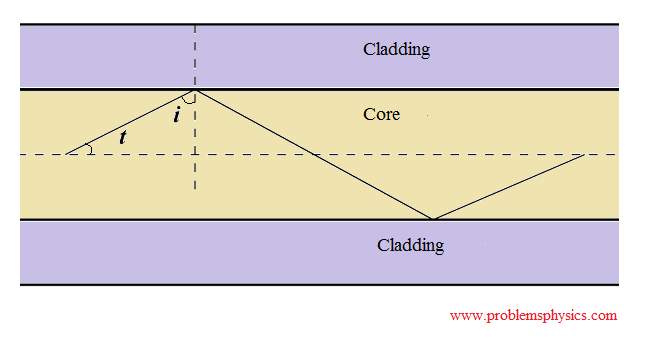Optical Fibers

# Optical Fibers

#### Reflection of Light Rays on a Reflecting Surface

Optical fiber systems are used in communication systems to transmit information using light as a carrier. Since light as an electromagnetic wave has frequencies of the order 1014 Hertz, very high rate of data may be transferred using optical fiber systems.
The optical fiber itself is used to guide light along a thin, long and flexible tube of glass called core with high refractive index, a cladding with lower refractive index surrounds the core so that total internal reflection takes place at the core-cladding boundary. Then the fiber itself is protected, against physical damages, by a jacket.

#### Principles of Optical Fibers: Total internal refractionAn optical fiber comprises a core and a cladding as shown in the diagram above. The core has refractive index n1 and the cladding has refractive index n2 such that n1 > n2 or n1 / n2 > 1.
Use Snell's law to write     n1 sin i = n2 sin r
The above equation may be written as :      n1 / n2 = sin r / sin i
Since n1 / n2 > 1 then sin r / sin i > 1
Angles i and r are less than 90 �, hence using sin r / sin i > 1 we can write
r / i > 1 or r > i.
If we start increasing i from 0, at some point r = 90 �. Let i = ic for r = 90 �
n1 sin iic = n2 sin 90�
ic is called the critical angle.
n1 sin ii = n2
sin ic = n2 / n1
Now we continue increasing i > ic
sin i > sin ic
sin i = n2 / n1 sin r and sin ic = n2 / n1
Substitute sin i by n2 / n1 sin r and sin ic by n2 / n1 in the above inequality to obtain
n2 / n1 sin r > n2 / n1
Simplify to obtain
sin r > 1
The sine of a real angle cannot be greater than 1. Hence angle r does not exist and therefore there is no refraction of light; it is all reflected back to the core. This is called total internal reflection. Therefore light is guided through successive reflections at the core-cladding boundary for very long distances with acceptable losses.

#### Comparison of optical fibers and copper cable

1) The carrier in optical fiber is light, which has a higher frequency, therefore the rate of information sent through optical fibers is much higher than that in cables.
2) The attenuation of signals in optical fibers is lower than in cables.
3) It is not easy to tap into an optical fiber and therefore information sent through optical fibers is more secure.
4) Optical fibers are lighter and are of smaller sizes than cables
5) Electromagnetic external signals do not affect signals in optical fibers.
6) Optical fibers are more expensive to make, maintain and protect.

#### Use of Optical Fibers

Optical fibers are used

1) in communication systems to carry digitally coded signals with high rate.
2) in medicine applied in endoscopy
3) as sensors to measure pressure, temperature
4) in seismic applications
5) in numerous underwater applications

Example 1In the optical fiber below, the core has a refractive index equal to 1.5 and a cladding of refractive index of 1.4.
a) What is the speed of light inside the core?
b) What is the critical angle at the core-cladding interface?
c) What is the maximum angle t that the rays leaving the source of light should make with the axis of the fiber so that total internal reflections takes place at the core cladding interface?

Solution
a)
Refractive index n1 of core is defined as: n1 = c / v, where c = 3�108 m/s is the speed of light in vacuum and v is the speed of in the core.
Hence v = c / n1 = 3�108 m/s / 1.5 = 2�108 m/s
b)
Critical angle ic is such that sin ic = n2 / n1 ic = arcsin ( n2 / n1 ) = arcsin (1.4 / 1.5) ≈ 69�
c)
In order to have total internal reflection, we need to have i > ic
i = 90 - t
Hence
90 - t > ic
t < 90 - ic
t < 21�
Maximum size of angle t must be smaller than 21� in order to have total internal reflection at the core-cladding interface.